C 语言指针详解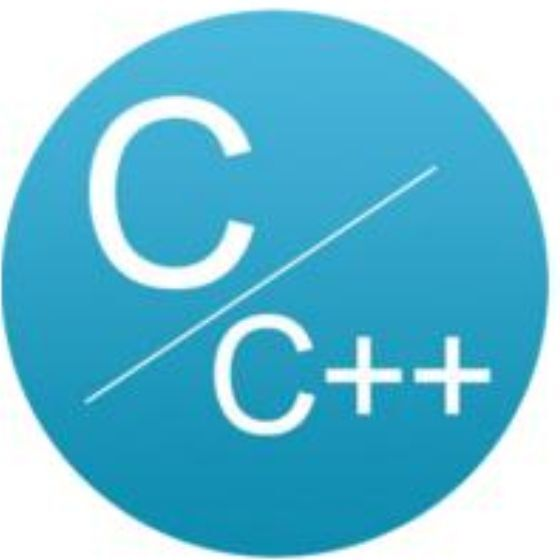C语言与CPP编程

﻿﻿

1为什么使用指针

• 使用指针型变量在很多时候占用更小的内存空间。

• 在数据传递时，如果数据块较大，可以使用指针传递地址而不是实际数据，即提高传输速度，又节省大量内存。

*(short*)&buf=DataId;
*(short*)&buf=DataType;
*(int*)&buf=DataValue;
• 数据转换，利用指针的灵活的类型转换，可以用来做数据类型转换，比较常用于通讯缓冲区的填充。

• 指针的机制比较简单，其功能可以被集中重新实现成更抽象化的引用数据形式

• 函数指针，形如: #define PMYFUN (void*)(int,int) ，可以用在大量分支处理的实例当中，如某通讯根据不同的命令号执行不同类型的命令，则可以建立一个函数指针数组，进行散转。

• 在数据结构中，链表、树、图等大量的应用都离不开指针。

2 指针是什么？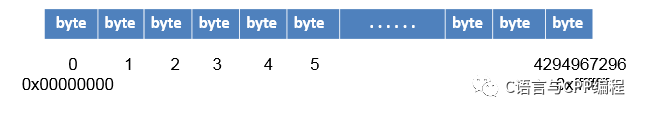﻿

#include <stdio.h>
int main(void)
{
char ch = 'a';
int num = 97;
printf("ch 的地址:%p\n",&ch);
//ch 的地址:00BEFDF7
printf("num的地址:%p\n",&num);
//num的地址:00BEFDF8
return 0;
}

﻿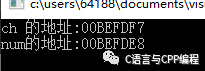﻿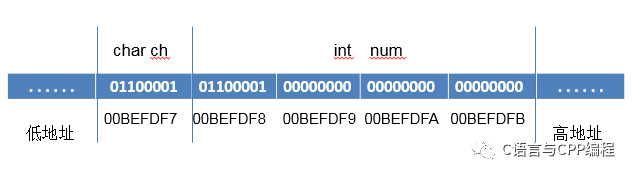指针变量 和 指向关系

#include <stdio.h>
int main(void)
{
int num = 97;
char ch = 'a';
int *p = & num;
int **pp = &p;
char *p1 = & ch;
printf("num 的地址:%p\n",&num);
printf("指针p的值:%p\n",p);
printf("指针p的地址:%p\n",&p);
printf("指针pp的值:%p\n",pp);
printf("ch 的地址:%p\n",&ch);
return 0;
}

﻿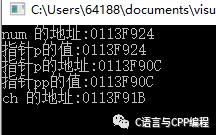• int型的num值为97占4个字节，内存地址为:0113F924char 型的ch('a')值为97占1个字节，内存地址为:0113F91B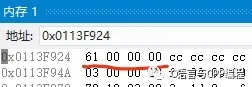int型占4个字节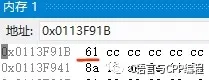char型占1个字节

• num的地址为：0113F924num的值为 97 ，指针 p 指向 num 的内存块，指针 p 地址为：0113F90Cp的内存保存的值就是num的地址0113F924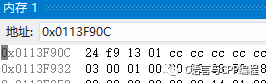0x0113F90C存储的内容为地址0113F924

• 指针变量 pp 指向 指针 p，指针 pp 内存值为 指针 p 的地址：0113F90C，形成了只想指针的指针。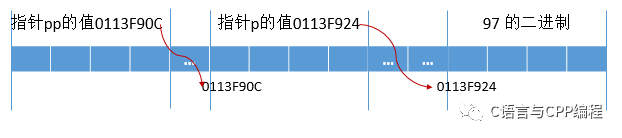定义指针变量

C语言中，定义变量时，在变量名 前 写一个 * 星号，这个变量就变成了对应变量类型的指针变量。必要时要加( ) 来避免优先级的问题。

int a ; //int类型变量 a
int *a ; //int* 变量a
int arr; //arr是包含3个int元素的数组
int (* arr ); //arr是一个指向包含3个int元素的数组的指针变量
int* p_int; //指向int类型变量的指针
double* p_double; //指向idouble类型变量的指针
struct Student *p_struct; //结构体类型的指针
int(*p_func)(int,int); //指向返回类型为int，有2个int形参的函数的指针
int(*p_arr); //指向含有3个int元素的数组的指针
int** p_pointer; //指向 一个整形变量指针的指针

取地址

int add(int a , int b)
{
return a + b;
}
int main(void)
{
int num = 97;
float score = 10.00F;
int arr = {1,2,3};
int* p_num = &num;
float* p_score = &score;
int (*p_arr) = &arr;
return 0;
}

• 数组名的值就是这个数组的第一个元素的地址。

• 函数名的值就是这个函数的地址。

• 字符串字面值常量作为右值时，就是这个字符串对应的字符数组的名称,也就是这个字符串在内存中的地址。

int add(int a , int b){
return a + b;
}
int main(void)
{
int arr = {1,2,3};
int* p_first = arr;
const char* msg = "Hello world";
return 0;
}

解地址

int main(void)
{
int age = 19;
int*p_age = &age;
*p_age = 20; //通过指针修改指向的内存数据
printf("age = %d",*p_age); //通过指针读取指向的内存数据
printf("age = %d",age);
return 0;
}

空指针

#ifdef __cplusplus
#define NULL 0
#else
#define NULL ((void *)0)
#endif

void*类型指针

void是一种特殊的指针类型，可以用来存放任意对象的地址。一个void指针存放着一个地址，这一点和其他指针类似。不同的是，我们对它到底储存的是什么对象的地址并不了解。

double a=2.3;
int b=5;
void *p=&a;
cout<<p<<endl; //输出了a的地址
p=&b;
cout<<p<<endl; //输出了b的地址
//cout<<*p<<endl;这一行不可以执行，void*指针只可以储存变量地址，不可以直接操作它指向的对象

数组和指针

• 同类型指针变量可以相互赋值，数组不行，只能一个一个元素的赋值或拷贝

• 数组在内存中是连续存放的，开辟一块连续的内存空间。数组是根据数组的下进行访问的。指针很灵活，它可以指向任意类型的数据。指针的类型说明了它所指向地址空间的内存。

• 数组所占存储空间的内存：sizeof（数组名） 数组的大小：sizeof（数组名）/sizeof（数据类型），在32位平台下，无论指针的类型是什么，sizeof（指针名）都是 4 ，在 64 位平台下，无论指针的类型是什么，sizeof（指针名）都是 8 。

• 数组名作为右值的时候，就是第一个元素的地址

int main(void)
{
int arr = {1,2,3,4,5};
int *p_first = arr;
printf("%d",*p_first); //1
return 0;
}
• 指向数组元素的指针 支持 递增 递减 运算。p= p+1意思是，让p指向原来指向的内存块的下一个相邻的相同类型的内存块。在数组中相邻内存就是相邻下标元素。

函数与指针

函数的参数和指针

C语言中，实参传递给形参，是按值传递的，也就是说，函数中的形参是实参的拷贝份，形参和实参只是在值上面一样，而不是同一个内存数据对象。这就意味着：这种数据传递是单向的，即从调用者传递给被调函数，而被调函数无法修改传递的参数达到回传的效果。

void change(int a)
{
a++; //在函数中改变的只是这个函数的局部变量a，而随着函数执行结束，a被销毁。age还是原来的age，纹丝不动。
}
int main(void)
{
int age = 60;
change(age);
printf("age = %d",age); // age = 60
return 0;
}

void change(int* pa)
{
(*pa)++; //因为传递的是age的地址，因此pa指向内存数据age。当在函数中对指针pa解地址时，
//会直接去内存中找到age这个数据，然后把它增1。
}
int main(void)
{
int age = 160;
change(&age);
printf("age = %d",age); // age = 61
return 0;
}

#include <stdio.h>
#include <stdlib.h>
#include <string.h>
void swap(int *,int *);
int main()
{
int a=5,b=10;
printf("a=%d,b=%d\n",a,b);
swap(&a,&b);
printf("a=%d,b=%d\n",a,b);
return 0;
}
void swap(int *pa,int *pb)
{
int t=*pa;*pa=*pb;*pb=t;
}

• 定义函数的形参必须为指针类型，以接收主调函数中传来的变量的地址；

• 调用函数时实参为变量的地址；

• 在被调函数中使用*间接访问形参指向的内存空间，实现修改主调函数中变量值的功能。

int GetMax(int a[],int n)
{
int max=a,i;
for(i=1;i<n;i++)
{
if(max<a[i]) max=a[i];
}
return max;
}
int GetMin(int a[],int n)
{
int min=a,i;
for(i=1;i<n;i++)
{
if(min>a[i]) min=a[i];
}
return min;
}
double GetAvg(int a[],int n)
{
double avg=0;
int i;
for(i=0;i<n;i++)
{
avg+=a[i];
}
return avg/n;
}

double Stat(int a[],int n,int *pmax,int *pmin)
{
double avg=a;
int i;
*pmax=*pmin=a;
for(i=1;i<n;i++)
{
avg+=a[i];
if(*pmax<a[i]) *pmax=a[i];
if(*pmin>a[i]) *pmin=a[i];
}
return avg/n;
}

函数的指针

returnType (*pointerName)(param list);

returnType 为函数返回值类型，pointerNmae 为指针名称，param list 为函数参数列表。参数列表中可以同时给出参数的类型和名称，也可以只给出参数的类型，省略参数的名称，这一点和函数原型非常类似。

#include <stdio.h>
//返回两个数中较大的一个
int max(int a, int b)
{
return a>b ? a : b;
}
int main()
{
int x, y, maxval;
//定义函数指针
int (*pmax)(int, int) = max; //也可以写作int (*pmax)(int a, int b)
printf("Input two numbers:");
scanf("%d %d", &x, &y);
maxval = (*pmax)(x, y);
printf("Max value: %d\n", maxval);
return 0;
}

﻿

结构体和指针

typedef struct
{
char name;
int age;
float score;
}Student;
int main(void)
{
Student stu = {"Bob" , 19, 98.0};
Student*ps = &stu;
ps->age = 20;
ps->score = 99.0;
printf("name:%s age:%d
",ps->name,ps->age);
return 0;
}

const 和 指针

• 指向常量的指针，值不能改变，指向可改变

• 常指针值能改变，指向不可改变

• 指向常量的常指针，都不能改变

#include <stdio.h>
int main()
{
// 1 可改变指针
const int a = 10;
int *p = &a;
*p = 1000;
printf("*p = %d\n", *p);
// 2 可改变指针
const b = 10;
int *pb = &b;
pb = p;
printf("*pb = %d\n", *pb);
// 3
const c = 10;
int * const pc = &c;
*pc = 1000;
//pc = pb;不能改变
//4
const d = 10;
const * int const pd = &d;
//*pd = 1000; 不能改变
printf("\n");
return 0;
}

深拷贝和浅拷贝

#include <iostream>
using namespace std;
class CopyDemo
{
public:
CopyDemo(int pa,char *cstr) //构造函数，两个参数
{
this->a = pa;
this->str = new char; //指针数组，动态的用new在堆上分配存储空间
strcpy(this->str,cstr); //拷贝过来
}
//没写，C++会自动帮忙写一个复制构造函数，浅拷贝只复制指针,如下注释部分
//CopyDemo(CopyDemo& obj)
//{
// this->a = obj.a;
// this->str = obj.str; //这里是浅复制会出问题，要深复制
//}
CopyDemo(CopyDemo& obj) //一般数据成员有指针要自己写复制构造函数，如下
{
this->a = obj.a;
// this->str = obj.str; //这里是浅复制会出问题，要深复制
this->str = new char;//应该这样写
if(str != 0)
strcpy(this->str,obj.str); //如果成功，把内容复制过来
}
~CopyDemo() //析构函数
{
delete str;
}
public:
int a; //定义一个整型的数据成员
char *str; //字符串指针
};
int main()
{
CopyDemo A(100,"hello!!!");
CopyDemo B = A; //复制构造函数，把A的10和hello!!!复制给B
cout <<"A:"<< A.a << "," <<A.str << endl;
//输出A:100，hello!!!
cout <<"B:"<< B.a << "," <<B.str << endl;
//输出B:100，hello!!!
//修改后,发现A,B都被改变，原因就是浅复制，A,B指针指向同一地方，修改后都改变
B.a = 80;
B.str = 'k';
cout <<"A:"<< A.a << "," <<A.str << endl;
//输出A:100，kello!!!
cout <<"B:"<< B.a << "," <<B.str << endl;
//输出B:80，kello!!!
return 0;
}

参考资料

C Primer Plus（第五版）中文版

https://www.cnblogs.com/lulipro/p/7460206.html

﻿评论